# Deeplearning.ai CNN week 3: Object detection

#### Object Localization

• Classification vs Localization vs Dectection?
• Localization -> return a bounding box of the object inside image
• Adding the bounding box coordinate variables into the target of the network.
• p_c is the probability of having an object
• b_x, b_y, b_h, b_w : bounding box information
• c_1, c_2, c_3 : the classes of pedestrian/car/motorcycle. 1 if it is.

#### Landmark Detection

• The same idea as object localization, the difference is returning the “point” instead of bounding box.
• Data label now in pair of x,y coordinates of landmarks which want to be learned. After passing through some ConvNet.
• Labels have to consitant over training images.

#### Object detection

• Create the training set by crop the object region + its label
• In testing phase, detection by sliding the windows(different size) over image → push to ConvNet → predict.
• The main disadvantage, many possible crop in the image in different scale→ computational cost. Running under ConvNet as take time to predict in one crop image → slow.

#### Convolutional Implementation Sliding Windows

• How to turn Fully connected layer to convolutional layers ?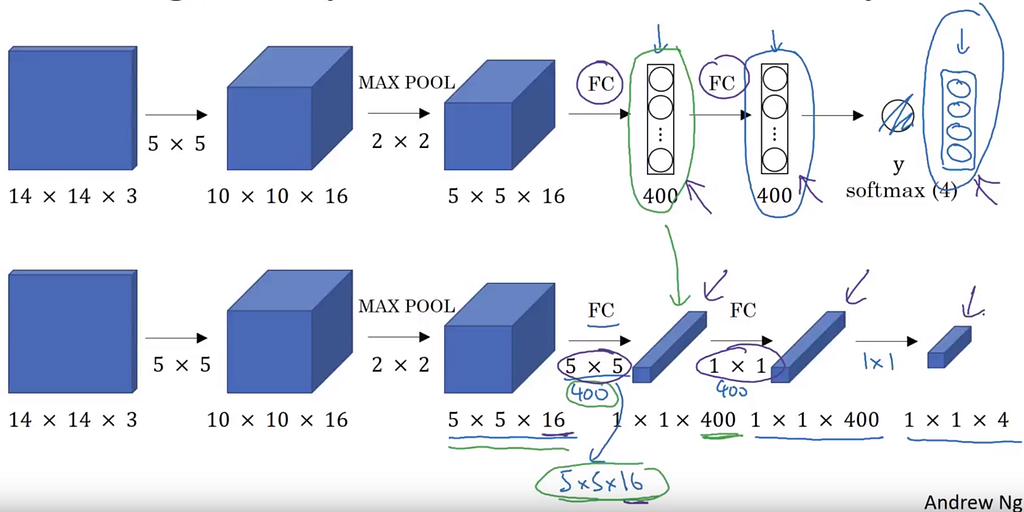Equivalent convolution based network (below) of the convo+fully connected network (above). Source: deeplearning.ai C4W3L04
• The main target is finding an appropriate way to keep the same network follow (input/output size) in each step, but not to use the fully connected layer anymore.
• Remember the “network inside network” : fully connected layer == 1×1 convolution. Then by using an “suitable” way, this target can be achieved.
• For the first fully connected layer of 400 nodes, due to its input is 5x5x16, we can use 400 convolution filters of the size 5x5x16. The result is 400 elements of the size 1×1 or 400 nodes.
• The next fully connected layers is rebuilt by using 1×1 convolution way, quite simple :).
• BUT the convolution filters ITSELF also sliding over regions of image to return the input. Then, the convolution is equivalent to the sliding window operator by an appropriate way.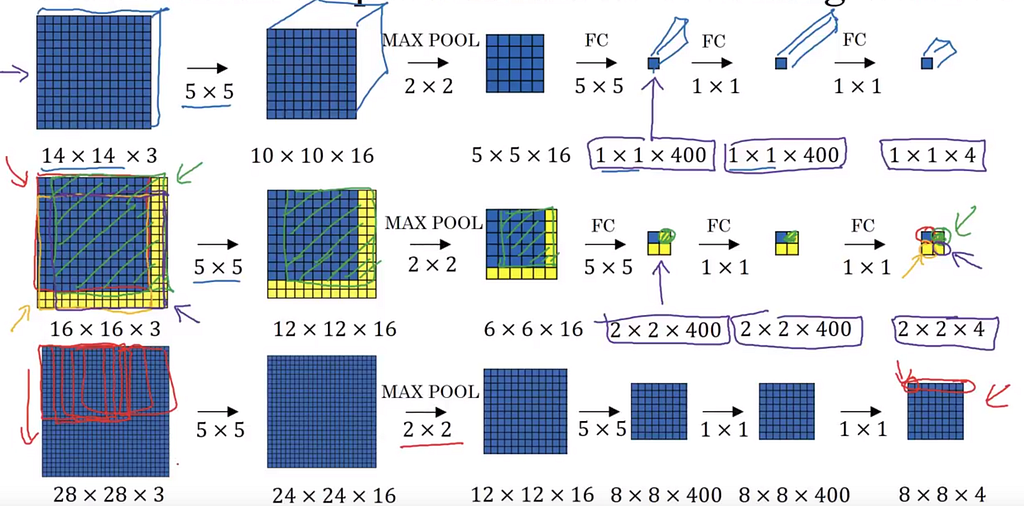1st row, trained model. 2nd, 3rd row, apply trained model in a new testing image. Source: deeplearning.ai C4W3L04
• For example, the test image 16x16x3 is 2 pixels more than the trained images. If putting them directly to the trained model, the final output is a 2x2x4 tensor where each 1x1x4 is corresponding the response of a region of 14x14x3 of the input testing image.

#### Bounding Box Predictions

• The size of sliding window is critical, as sequentially capture a region by region, there are no 100% chance to catch an object at a certain window size.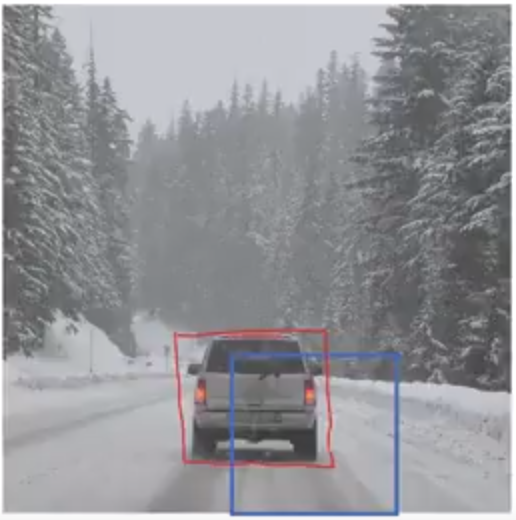Sliding window behaviour is not like our desire. Source: deeplearning.ai C4W3L05
• YOLO algorithm (you only look one time)
• For example: for an image of 100×100, divide into 3×3 grid, for the training data, each grid rectangle need to be labeled as “y” of 8 variables.
• p_c: probability response of having object or not
• b_x, b_y : the coordinate of the center point of the object bounding box.
• b_h, b_w: the height and weight of the bounding box
• c_1, c_2, c_3: binary respone of each label class.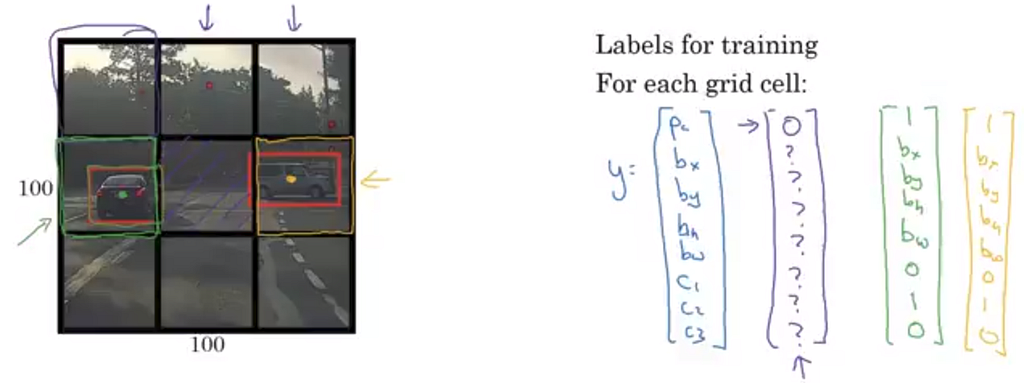Grid training data definition. Colors of right vectors are corresponding to the left gird area. Source: deeplearning.ai C4W3L05
• Then the output of this YOLO is a 3x3x8 tensor. Using the deep learning framework as usual, just modify the way of output.
• It is image classification + localization + convolutional implementation.
• Encode b_x, b_y, b_h, b_w information. By the fraction of it over the regional box.

#### Intersection over Union

• One object can be found in many boxes, causing the overlapping of object recognize.
• It is the size of the intersection / the union

#### Nonmax Suppression

• One object can be detected multiple times. Need to be clean up for the final result.
• Take the bounding box with the highest probability, get rid the rest of lower probability.
• The algorithm

#### Anchor Boxes

• What about overlapping objects ? How can recoginize multiple things in the same grid box?
• Predefine anchor boxes, each box for a type of shape, replicated the same 8 variable label structure into “y” more.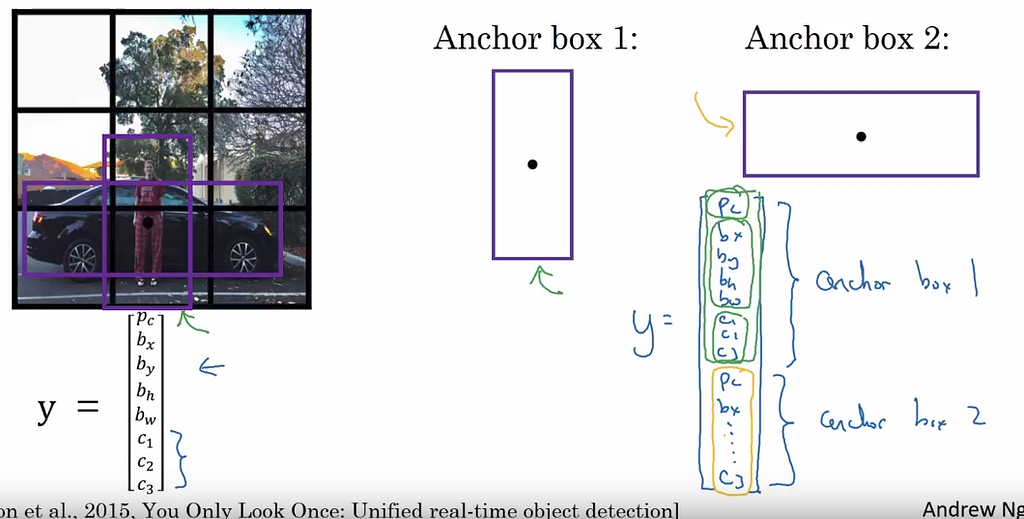Anchor box 1 for pedestrian and 2 for the car.Source: deeplearning.ai C4W3L08
• Algorithm with 2 anchor boxes: Each object in training image is assigned to the grid cell that contains object’s midpoint and anchor box for the grid cell with highest IoU.
• Choosing how many type of anchor boxes in term of shape and number is tough. Almost by human prior.
• Luckily, it is rarely happen that two objects appear in a grid cell IF the grid is 19×19 for 100×100 image size.
• Can use K-mean algorithm to cluster all of anchor box and finallize them later.

#### YOLO algorithm

• Putting all above components together. For example: classify three objects: car, pedestrian, motorcycle.
• There are two kind of anchor boxes: the red bounding box of the car is more IoU with the 2nd anchor box, then the label for the grid containing car as the above image (no information for the first 8 variables)
• Making the prediction